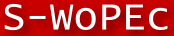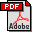Scandinavian Working Papers in Economics

# No 345: Regularized Conditional Estimators of Unit Inefficiency in Stochastic Frontier Analysis, with Application to Electricity Distribution Market

Zangin Zeebari (), Kristofer Månsson (), Pär Sjölander () and Magnus Söderberg ()
Zangin Zeebari: Jönköping University
Kristofer Månsson: Jönköping University
Pär Sjölander: Jönköping University
Magnus Söderberg: The Ratio Institute, Postal: The Ratio Institute, P.O. Box 5095, SE-102 42 Stockholm, Sweden

Abstract: The practical value of Stochastic Frontier Analysis (SFA) is positively related to the level of accuracy at which it estimates unit-specific inefficiencies. Conventional SFA unit inefficiency estimation is based on the mean/mode of the inefficiency, conditioned on the estimated composite error. This approach shrinks the inefficiency towards its mean/mode, which generates a distribution that is different from the distribution of the unconditional inefficiency; thus, the accuracy of the estimated inefficiency is negatively correlated with the distance the inefficiency is located from its mean/mode. We propose a regularized estimator based on Bayesian risk (expected loss) that restricts the unit inefficiency to satisfy the underlying theoretical mean and variation assumptions. We analytically investigate some properties of the maximum a posteriori probability estimator under mild assumptions and derive a regularized conditional mode estimator for three different inefficiency densities commonly used in SFA applications. Extensive simulations show that, under common empirical situations, e.g., regarding sample size and signal-to-noise ratio, the regularized estimator outperforms the conventional (unregularized) approach when the inefficiency is greater than its mean/mode. With real data from electricity distribution sector in Sweden, we demonstrate that the conventional conditional estimators and our regularized conditional estimators give substantially different results for highly inefficient companies.

JEL-codes: C21; D24; L94

32 pages, March 24, 2021

Full text files

`ratio-working-paper-no.-345.pdfFull text`

Questions (including download problems) about the papers in this series should be directed to Martin Korpi ()
Report other problems with accessing this service to Sune Karlsson ().